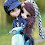## Wednesday, January 28, 2015

### Interference Fringe shift and Problems

Problem and solution

In the double slit experiment when a monochromatic source of wavelength lambda is used, the intensity of the light at a point on the screen for a path difference equal to the wavelength of the light is equal to K units. What is the intensity of the light at a point on the screen where the path difference is one third of the wavelength of the light?

When the path difference between the two sources is lambda, the resultant intensity is maximum. When the path difference is one third of the wavelength of the light we can calculate the corresponding phase difference. By substituting this value of the phase difference in the equation further resultant intensity we can get the value of resultant intensity as shown below.

Fringe visibility

Fringe visibility is the measure of comparison of the bright spots with respect to dark spots. We can write a small mathematical equation for it as shown below.

Fringes shift when a slab is placed in the path of the Ray

When a transparent sheet of thickness t is introduced in the path of the interfering wave, the interference pattern on the screen will shift to a different location.

We know that refractive index can be written like the ratio of apparent thickness the real thickness. Therefore because of the placing of the medium, there is a change in the path of the light as shown below. We can further simplify and identify the shift generated by the slab as shown below.

Problem and solution

In the double slit experiment how many maximums can be obtained on a screen when a wavelength of light 2000 Å is used and the distance between the slits is  7000 Å.

We can write the condition for the path difference as the integral multiples of wavelength of the light for the constructive interference. Basing on this condition we can solve the problem as shown below.

Problem and solution

In the double slit experiment when a monochromatic source of wavelength lambda is used, the intensity of the light at a point on the screen for a path difference equal to the wavelength of the light is equal to K units. What is the intensity of the light at a point on the screen where the path difference is one third of the wavelength of the light?

When the path difference between the two sources is lambda, the resultant intensity is maximum. When the path difference is one third of the wavelength of the light we can calculate the corresponding phase difference. By substituting this value of the phase difference in the equation further resultant intensity we can get the value of resultant intensity as shown below.

Problem and solution

In double slit experiment intensity at a point these one by fourth of the maximum intensity. What is the angular position of this point on the screen?

We can calculate the phase difference between the two points to have intensity one by fourth of the maximum intensity. It is found that the phase difference is 120°. By calculating the corresponding path difference and writing in the appropriate formula we can get the location of the point on the screen as shown below.

Problem and solution

In double slit experiment two slits are separated by 0.25 cm and the screen is it 120 cm from them. The slits are eliminated by light of wavelength of 600 nm. What is the distance between the first point on the screen from the central maximum where the intensity is a 75% of the maximum intensity?

As the intensity at a given point these three by fourth of the maximum intensity, we can calculate the phase difference between the two points as 60° as shown below. We can further calculate the path difference and by substituting the value of the path difference on the location of the point, we can calculate it as shown below.

Problem and solution

A double slit experiment is performed in a liquid. The 10th bright fringe in liquid lies where the six the dark fringe lies in vacuum. What is the refractive index of the liquid?

Basing on the mathematical equations that we have derived, we can identify the position of the bright spot as well as the dark spot. By substituting the given values in the problem we can get the answer as shown.

The attached paper is having one more problem and the detailed solution is also given. For any further clarifications it can comment at the end of the post.

Related Posts

#### 1 comment:

1.Handwritting is main drawback ! I like everything other ! Nice !!!